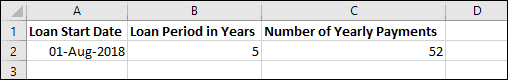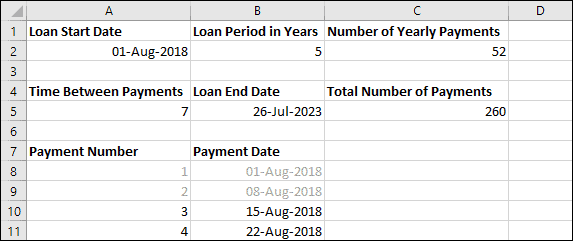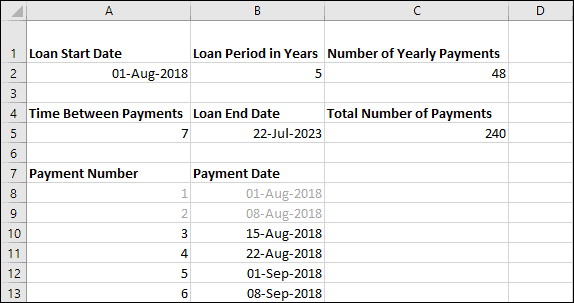# Calculating Loan Payment Dates

Loans are typically paid over several payments, sometimes monthly or weekly. It’s not unusual to need to calculate the dates that each of the payments will be due.

Calculating loan payment dates can be a rather difficult task to do accurately, because of the inconsistent nature of dates.

If you want to model a loan that spans a year and is paid weekly, adding 7 days between payments will not provide completely accurate results, because there are 365 days in a year and 52 7-day weeks only comprise 364 days. With the addition of leap years, things get even more complicated!

You can create a system whereby you can split the loan over any number of periods, but it may not tie up with weeks and months as neatly as you’d like. To do this, you’ll need to use the DATEYEARMONTHDAY and ROUND functions, along with an understanding of how dates are stored as serial numbers in Excel.

Date serial numbers are explain in depth in our Essential Skills Books and Videos.
Advanced functions such as DATE are fully explained in our Expert Skills Books and Videos.

## A formula to split a loan over any number of periods

Here is an example workbook.In this example you have a 5 year loan that is paid weekly (52 payments per year).

In order to calculate how much time should pass between each payment, you’ll first need to calculate the number of days that the loan spans. You can do this by using the DATE function to get the projected end date and then subtracting the start date. For example:

=DATE(YEAR(A2)+B2,MONTH(A2),DAY(A2))-A2

In your example the start date is 01/08/2018 and the loan period is 5 years, so the result will be:

=”01 Aug 2023″-“01 Aug 2018” = 1826

To get the number of days between periods, you just need to divide the number of days by the number of expected periods. In this example you’re using 52 periods per year, so the calculation will be:

1826/(52 * 5) = 7.023

In the context of the workbook, this formula is:

=(  DATE(YEAR(A2)+B2,MONTH(A2),DAY(A2))-A2  )/(C2*B2)

7.023 represents 7 days and 33 minutes. You could leave this number as-is if you wanted to space the payments completely evenly throughout the period (ie. a payment is made every 7 days and 33 minutes) or you could round this number to 0 decimal places to get the number of whole days. You can round the number to zero decimal places using the ROUND function:

=ROUND((DATE(YEAR(A2)+B2,MONTH(A2),DAY(A2))-A2)/(C2*B2),0)

Now that you know the number of days between each payment, it’s simply a matter of adding this number of days to the start date until you have all of the loan payments.Because we rounded off the 33 minutes from every payment, the loan ends about 6 days earlier than projected (33 minutes * 260 periods = 8580 minutes = 143 hours = 5.9 days).

Removing the rounding from the formula in cell A5 returns the end date to the expected 01 Aug 2023, but some of the payment dates will have changed as a result. It’s up to you which way you prefer to calculate the dates.

You should be able to use this formula to calculate loan payment dates for a loan of any length, paid over any number of periods.

## Sample workbook

You can download the sample workbook to see the formulas in action.## A more complex calculation

The customer who first approached us with this question wanted to calculate his loan payments in a more complex way. He wanted 4 weekly payments per month with the first monthly payment always taking place on the 1st of the month.

This means that there will be a variable time between payments at the end of the month, but you can calculate the correct dates by using the MOD function.

MOD is covered in depth in our Expert Skills Books and E-books.

The formula you will need is:

=IF(MOD(A9,4)=1,DATE(YEAR(B8),MONTH(B8)+1,1),B8+\$A\$5)

MOD returns the remainder after dividing. In this case it is dividing the payment number by 4, meaning it will only return 1 for every 4 payments – exactly what is needed in this case.

The IF function is used to move to the next month every 4 payments and to add the standard time between payments otherwise.

As before, you can download a sample workbook showing this in action.Scroll to Top